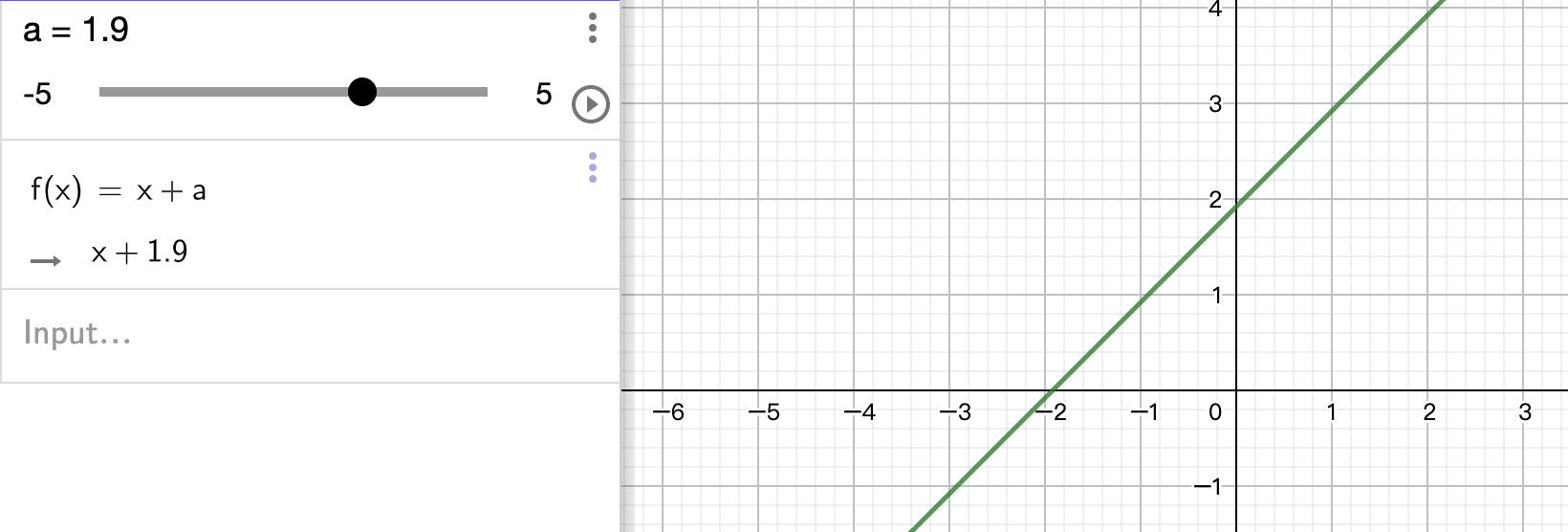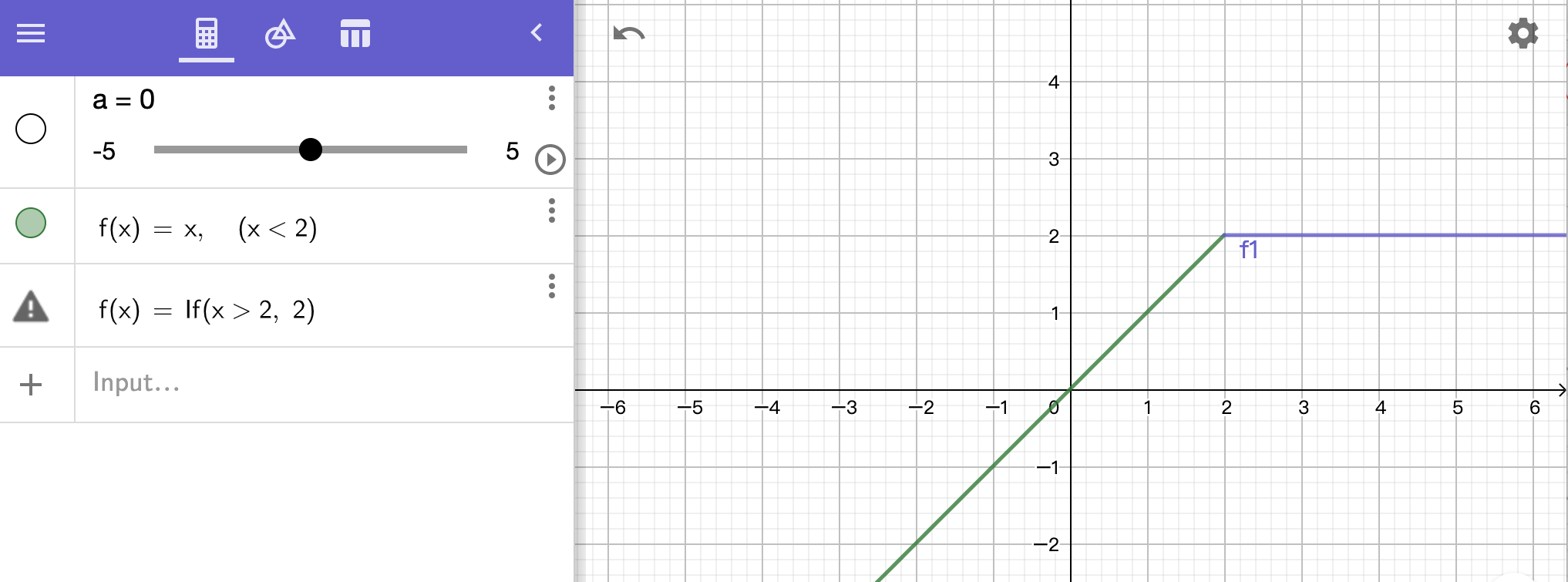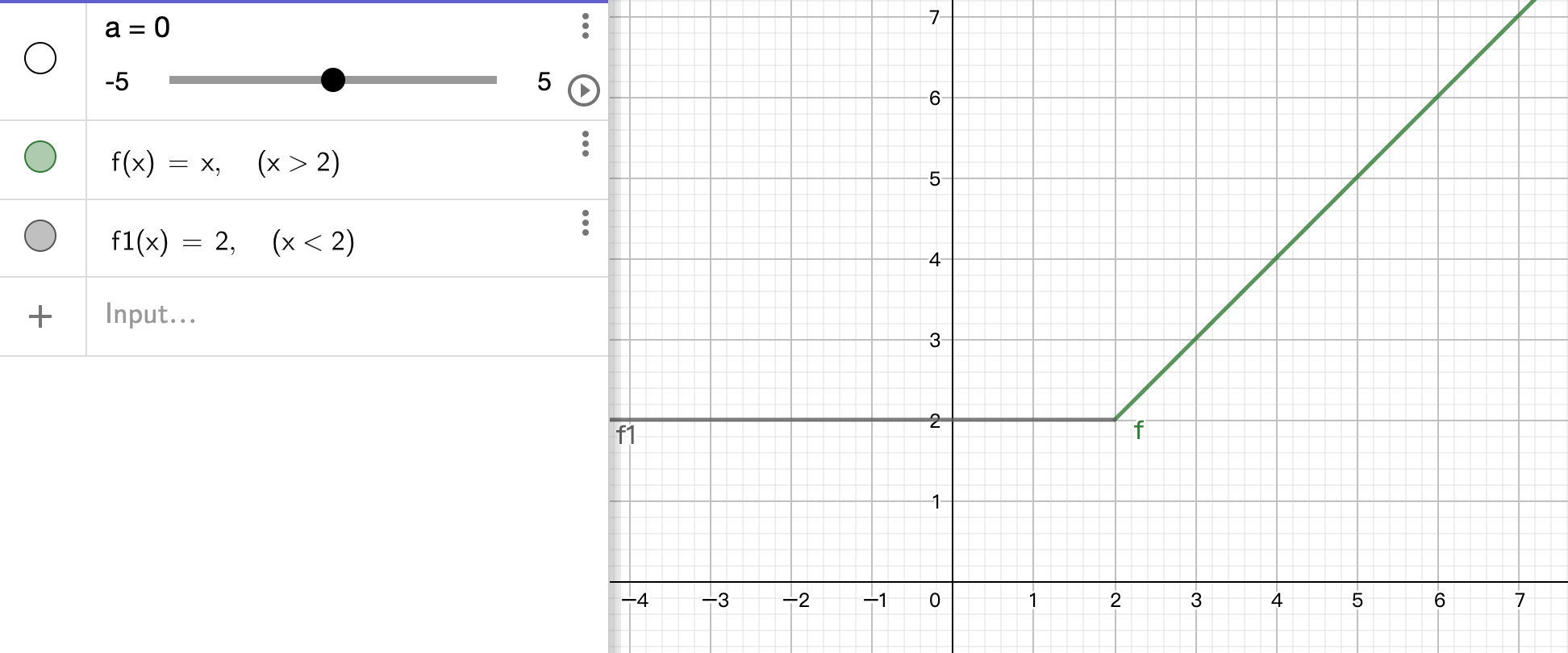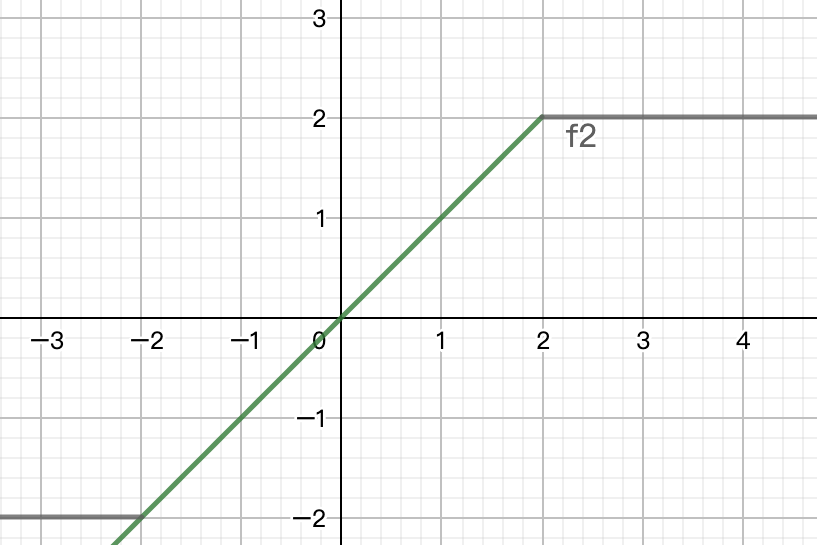signed

QiShunwang

“诚信为本、客户至上”

# AtCoder Beginner Contest 196 题解报告，AC了 A~E，缺 F

2021/3/21 11:58:17   来源：

# AtCoder Beginner Contest 196

https://atcoder.jp/contests/abc196/tasks。

## A - Difference Max

https://atcoder.jp/contests/abc196/tasks/abc196_a。

#include <bits/stdc++.h>
using namespace std;
int main() {
int a,b,c,d;
cin>>a>>b>>c>>d;
int x=max(a,b);
int y=min(c,d);
cout<<x-y<<"\n";
return 0;
}

## B - Round Down

https://atcoder.jp/contests/abc196/tasks/abc196_b。

#include <bits/stdc++.h>
using namespace std;
int main() {
string s;
cin>>s;
for (int i=0; i<s.length(); i++) {
if (s[i]!='.') {
cout<<s[i];
} else {
break;
}
}
cout<<"\n";
return 0;
}

## C - Doubled

https://atcoder.jp/contests/abc196/tasks/abc196_c。

#include <iostream>
#include <string>
using namespace std;
typedef long long ll;
int main() {
ll n;
cin>>n;
ll ans=0;
for (ll i=1; ; i++) {
//将 i 合并
string s=to_string(i)+to_string(i);
ll t=stoll(s);
if (t>n) {
cout<<i-1<<"\n";
return 0;
}
}
return 0;
}

#include <iostream>
#include <cstdio>
#include <string>
using namespace std;
typedef long long ll;

ll to_number(string s) {
ll ans=0;
for (int i=0; i<s.length(); i++) {
ans = ans*10+s[i]-'0';
}
return ans;
}

int main() {
ll n;
cin>>n;
ll ans=0;
for (ll i=1; ; i++) {
//将 i 合并
char buf;
sprintf(buf, "%d%d", i, i);
string s=buf;
ll t=to_number(s);
if (t>n) {
cout<<i-1<<"\n";
return 0;
}
}
return 0;
}

## D - Hanjo

https://atcoder.jp/contests/abc196/tasks/abc196_d。

#include <iostream>
#include <cstdio>
#include <string>
using namespace std;

int h,w;
int ans=0;

/*

*/
void dfs(int i, int bit, int a, int b) {
if (i==h*w) {
ans++;
return;
}
if (bit & 1<<i) {
dfs(i+1, bit, a, b);
return;
}
if (b) {
dfs(i+1, bit|1<<i, a, b-1);
}
if (a) {
if (i%w!=w-1 && (~bit&1<<(i+1))) {
dfs(i+1, bit|1<<i|1<<(i+1), a-1, b);
}
if (i+w<h*w) {
dfs(i+1, bit|1<<i|1<<(i+w), a-1, b);
}
}
}
int main() {
int a,b;
cin>>h>>w>>a>>b;
dfs(0,0,a,b);
cout<<ans<<"\n";
return 0;
}

## E - Filters

。我们知道这是一个函数族，是与 平行的函数族。该函数的图像如下图，。当  的时候，是一个平行于 x 轴的直线；当  时候，是一个斜率为 1 的直线。如下图。同理，如下图#include <iostream>

using namespace std;
typedef long long ll;
const ll INF=2e18;

ll clamp(ll x, ll min, ll max) {
if (x>max) {
return max;
}
if (x<min) {
return min;
}
return x;
}

int main() {
ll n;
cin>>n;

ll low=-INF;
ll high=INF;
ll bias=0;

for (ll i=1; i<=n; i++) {
ll a,t;
cin>>a>>t;
if (1==t) {
low+=a;
high+=a;
bias+=a;
} else if (2==t) {
low=max(low, a);
high=max(high,a);
} else {
low=min(low, a);
high=min(high, a);
}
}

ll q;
cin>>q;
for (ll i=1; i<=q; i++) {
ll x;
cin>>x;
cout<<clamp(x+bias, low, high)<<"\n";
}

return 0;
}